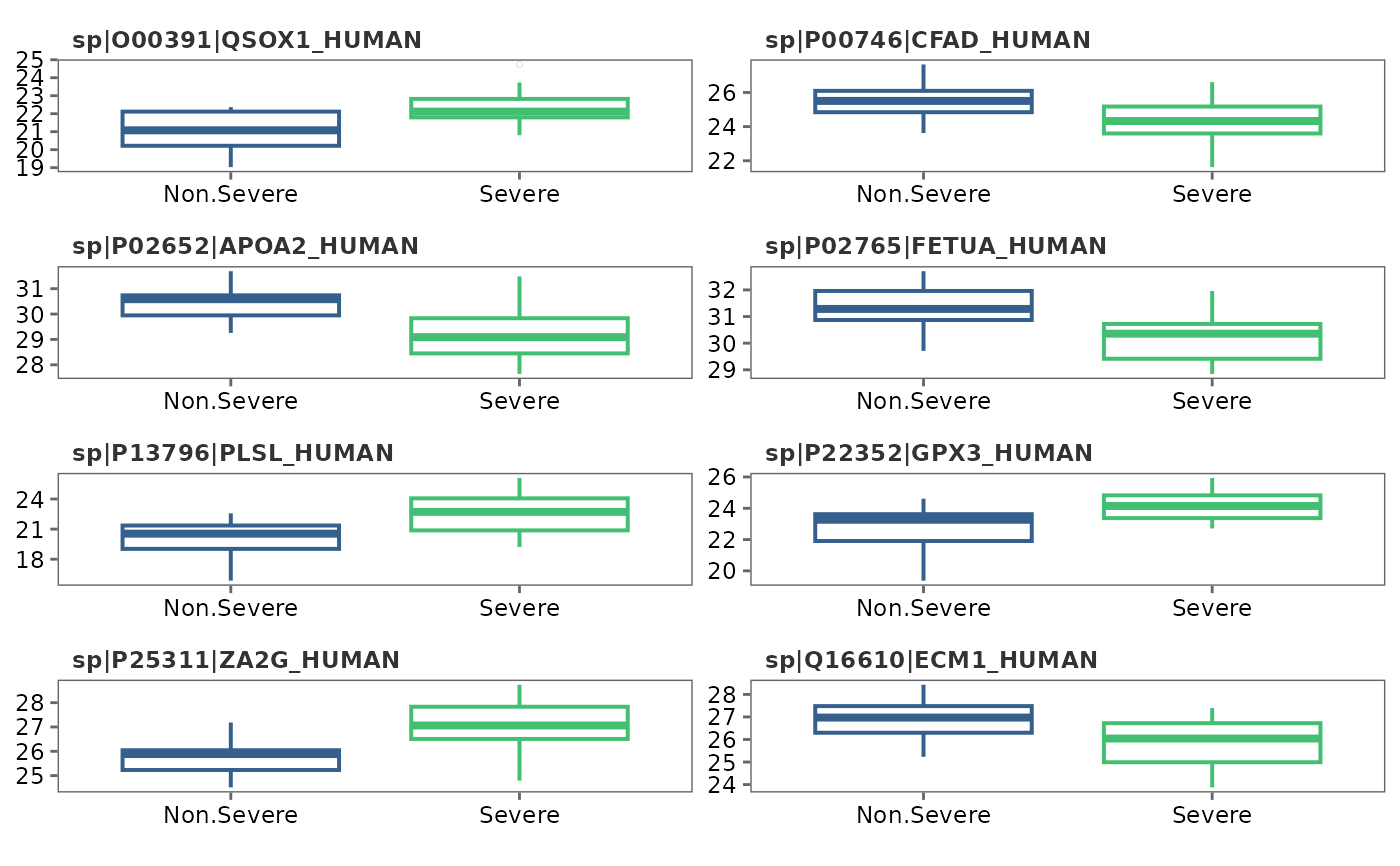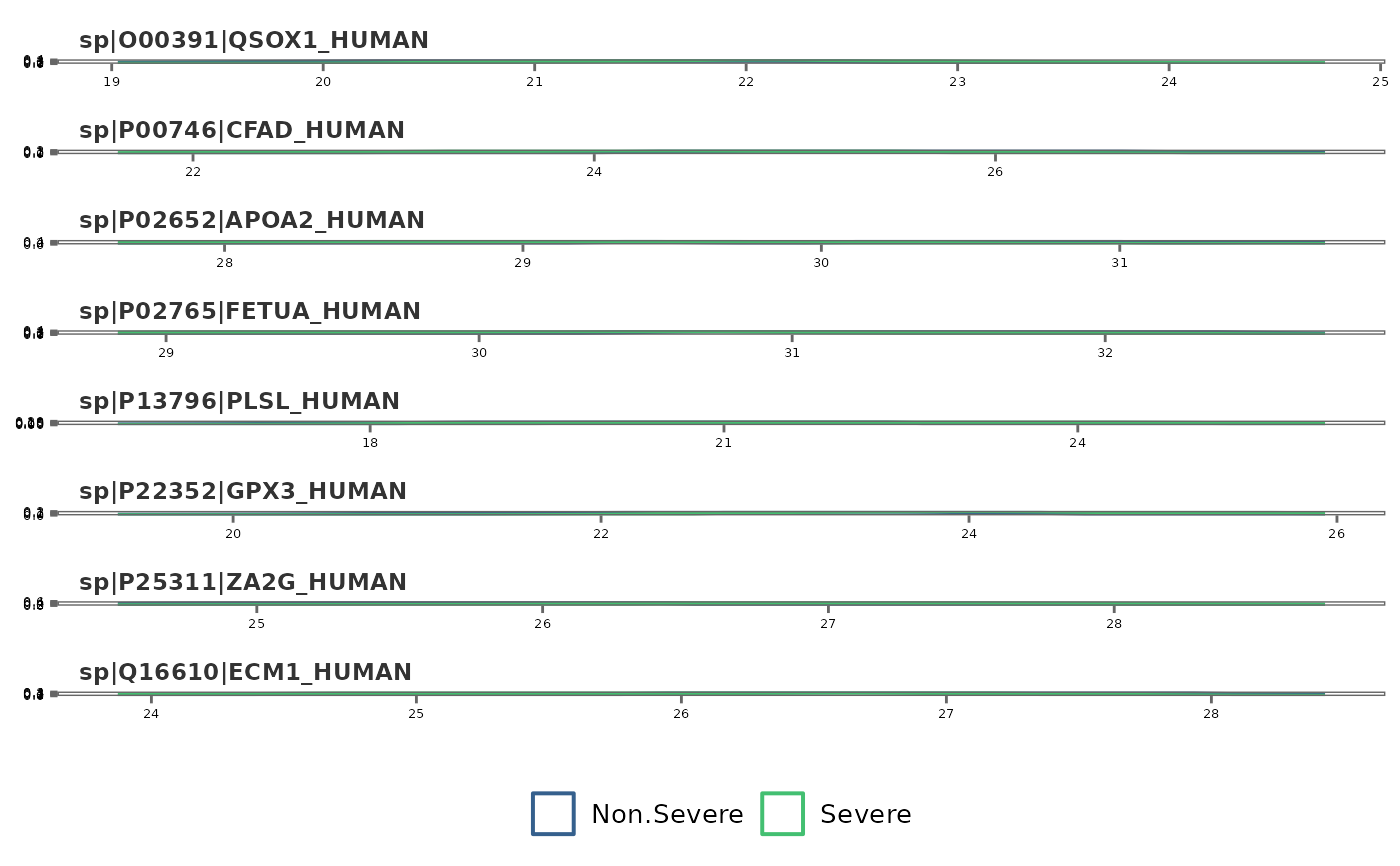This function visualizes protein intensity differences among conditions (classes) using box plots or density distribution plots.

## Usage

feature_plot(
model_df,
type = "box",
text_size = 10,
palette = "viridis",
n_row,
n_col,
save = FALSE,
file_path = NULL,
file_name = "Feature_plot",
file_type = "pdf",
dpi = 80,
plot_width = 7,
plot_height = 7
)

## Arguments

model_df

A model_df object from performing pre_process.

type

Type of plot to generate. Choices are "box" or "density." Default is "box."

text_size

Text size for plot labels, axis labels etc. Default is 10.

palette

Viridis color palette option for plots. Default is "viridis". See viridis for available options.

n_row

Number of rows to print the plots.

n_col

Number of columns to print the plots.

save

Logical. If TRUE saves a copy of the plot in the directory provided in file_path.

file_path

A string containing the directory path to save the file.

file_name

File name to save the plot. Default is "Feature_plot."

file_type

File type to save the plot. Default is "pdf".

dpi

Plot resolution. Default is 80.

plot_width

Width of the plot. Default is 7.

plot_height

Height of the plot. Default is 7.

## Value

A ggplot2 object

## Details

This function visualizes condition-wise differences in protein intensity using boxplots and/or density plots.

• pre_process, rem_feature

## Author

Chathurani Ranathunge

## Examples


## Create a model_df object with default settings.
covid_model_df <- pre_process(covid_fit_df, covid_norm_df)
#> Total number of differentially expressed proteins (8) is less than n_top.
#> None of the proteins show high pair-wise correlation.
#>
#> No highly correlated proteins to be removed.

## Feature variation - box plots
feature_plot(covid_model_df, type = "box", n_row = 4, n_col = 2)
#> Using condition as id variables## Density plots
feature_plot(covid_model_df, type = "density")
#> Using condition as id variables## Change color palette
feature_plot(covid_model_df, type = "density", n_row = 4, n_col = 2, palette = "rocket")
#> Using condition as id variables